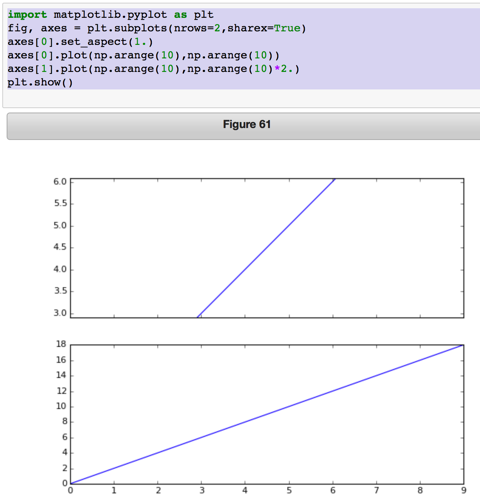# sharex with one figure aspect = 1.0

Hi Eric,

import matplotlib.pyplot as plt
fig, axes = plt.subplots(nrows=2,sharex=True)
axes.set_aspect(1.)
axes.plot(np.arange(10),np.arange(10))
axes.set_ylim([0,24])
axes.set_xlim([0,12])
axes.plot(np.arange(10),np.arange(10)*2.)
plt.show()

does not work as I’d expect. axes's ylim gets changed so that the line is no longer viewable (= 10-14). In my opinion, the two calls should work the same, except in the second case, axes's xlim should be 0-12.

If you leave out the set_ylim call, it works. Given that you have specified set_ylim[0, 24], how is mpl supposed to know what ylim range you really want, when the axis constraint means it can only plot a small part of the specified range?

It doesn’t for me:

import matplotlib.pyplot as plt
fig, axes = plt.subplots(nrows=2,sharex=True)
axes.set_aspect(1.)
axes.plot(np.arange(10),np.arange(10))
axes.plot(np.arange(10),np.arange(10)*2.)
plt.show()

still curtails the y limit in axes, in my case from ~2.9 to ~6.1 (see attached).

Basically, you have set up conflicting constraints, and mpl failed to resolve the conflict the way you think it should have. Maybe that could be improved, but I warn you, it’s a tricky business. Squeeze in one place and things pop out somewhere else.

I’m not clear what the conflicting constraints are. There seems to be an unspoken one that sharex=True means the physical size of the axes must be the same. That constraint doesn’t exist if sharex=False, and set_aspect() works as expected. I’m questioning the unspoken constraint, and questioning why set_aspect() (or looking at the code apply_aspect()) needs to know about shared axes at all. No doubt there is a use case I’m missing…

I can see the issue if we think setting aspect ratios should not change the size of the axes, because changing the aspect ratio changes the data limits and then you have a problem checking all the shared axes to see which one has the largest data limits. Its particularly problematic because I think that apply_aspect() is only called at draw()-time. That seems a hard problem, but I’m not sure what the use case is for it, so I have trouble wrapping my head around it.

Thanks, Jody···

Jody Klymak

http://web.uvic.ca/~jklymak/

Hi Eric,

import matplotlib.pyplot as plt
fig, axes = plt.subplots(nrows=2,sharex=True)
axes.set_aspect(1.)
axes.plot(np.arange(10),np.arange(10))
axes.set_ylim([0,24])
axes.set_xlim([0,12])
axes.plot(np.arange(10),np.arange(10)*2.)
plt.show()

does not work as I'd expect. axes's ylim gets changed so that the
line is no longer viewable (= 10-14). In my opinion, the two calls
should work the same, except in the second case, axes's xlim
should be 0-12.

If you leave out the set_ylim call, it works. Given that you have
specified set_ylim[0, 24], how is mpl supposed to know what ylim range
you really want, when the axis constraint means it can only plot a
small part of the specified range?

It doesn't for me:

import matplotlib.pyplot as plt
fig, axes = plt.subplots(nrows=2,sharex=True)
axes.set_aspect(1.)
axes.plot(np.arange(10),np.arange(10))
axes.plot(np.arange(10),np.arange(10)*2.)
plt.show()

still curtails the y limit in axes, in my case from ~2.9 to ~6.1 (see
attached).

Jody, you told it you want x to go from 0 to 12, and have an aspect ratio of 1. It is doing exactly that. Are you expecting it to override your xlim specification?

Basically, you have set up conflicting constraints, and mpl failed
to resolve the conflict the way you think it should have. Maybe that
could be improved, but I warn you, it's a tricky business. Squeeze in
one place and things pop out somewhere else.

I'm not clear what the conflicting constraints are. There seems to be
an unspoken one that sharex=True means the physical size of the axes
must be the same. That constraint doesn't exist if sharex=False, and
set_aspect() works as expected. I'm questioning the unspoken
constraint, and questioning why set_aspect() (or looking at the code
apply_aspect()) needs to know about shared axes at all. No doubt there
is a use case I'm missing...

Yes, sharex=True means the x-axis is identical. It's shared. That's just what it means, and what it has always meant. That's what it is for--to lock together the x axes of two or more Axes.

I *can* see the issue if we think setting aspect ratios should *not*
change the size of the axes, because changing the aspect ratio changes
the data limits and then you have a problem checking all the shared axes
to see which one has the largest data limits. Its particularly
problematic because I think that apply_aspect() is only called at
draw()-time. That seems a hard problem, but I'm not sure what the use
case is for it, so I have trouble wrapping my head around it.

The use case for what--calling apply_aspect at draw time? That's the only time it knows everything it needs to know in the general case, when there might be pan/zoom/reshape events.

Eric

···

On 2015/04/08 11:15 AM, Jody Klymak wrote:

Thanks, Jody

--
Jody Klymak
http://web.uvic.ca/~jklymak/

------------------------------------------------------------------------------
BPM Camp - Free Virtual Workshop May 6th at 10am PDT/1PM EDT
Develop your own process in accordance with the BPMN 2 standard
Learn Process modeling best practices with Bonita BPM through live exercises
http://www.bonitasoft.com/be-part-of-it/events/bpm-camp-virtual- event?utm_
source=Sourceforge_BPM_Camp_5_6_15&utm_medium=email&utm_campaign=VA_SF

_______________________________________________
Matplotlib-users mailing list
Matplotlib-users@lists.sourceforge.net
https://lists.sourceforge.net/lists/listinfo/matplotlib-users